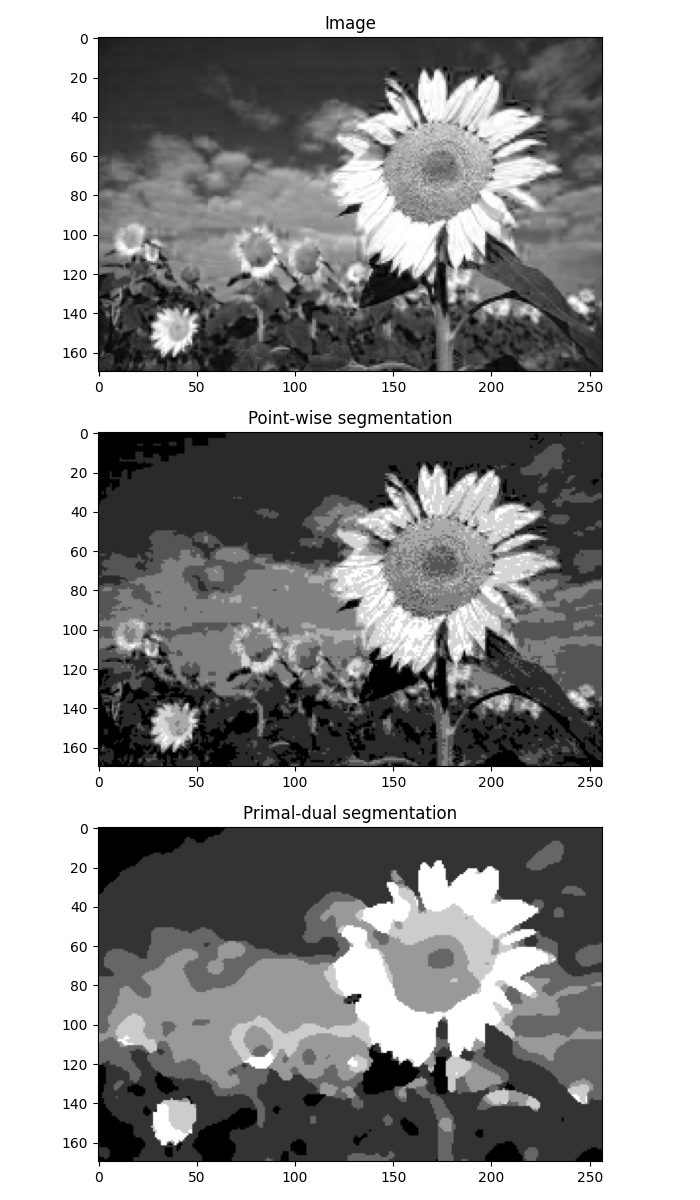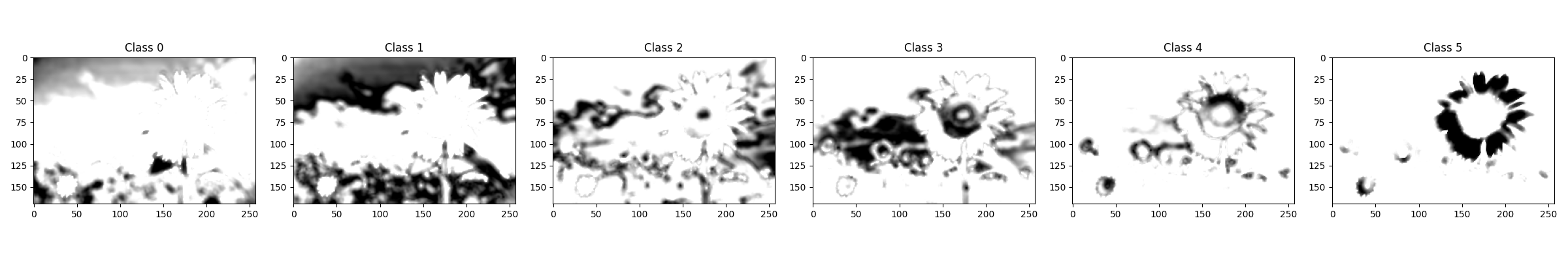# Image segmentation#

This tutorial shows how we can use the pyproximal.optimization.primaldual.PrimalDual solver to perform image segmentation. A modified version of such a solver that can directly used for segmentation is provided by pyproximal.optimization.segmentation.Segment.

The problem statement is as follows: given an image $$\mathbf{x}$$, we want to divide the image into $$N_{cl}$$ pairwise disjoint regions such that we jointly minimize the difference between the image values and their assigned class values for each image pixel as well as the total interface between the sets.

See Notes in pyproximal.optimization.segmentation.Segment for a more precise mathematical description of the problem.

import numpy as np
import matplotlib.pyplot as plt

import pyproximal

plt.close('all')


Let’s start loading an image and choosing a single channel (we will work with gray scale image in this tutorial)

im = plt.imread('../testdata/sunflower.png')
im = im[::2, ::2, :3]
ny, nx, _ = im.shape

ig = im[..., 0] # use grayscale


We can now define a number of classes we want to segment the image in

ncl = 6

cl = np.linspace(ig.min(), ig.max(), ncl+1)
dcl = cl - cl
cl = (cl + dcl/2)[:-1]


The simplest segmentation we can do is to simply assign each pixel to its closest class. This is equivalent to solving our cost function and ignoring the term that minimizes the total interface between the sets. As a result our segmentation boundaries will be very crisp.

ic = np.floor(ig / dcl).astype(np.int64)


On the other hand, we can choose to get much smoother boundaries if we use our primal dual solver.

sigma = 10.
alpha = 1.
isegcl, iseg = pyproximal.optimization.segmentation.Segment(ig, cl,
sigma, alpha,
niter=10,
kwargs_simplex=dict(
maxiter=20,
engine='numba',
call=False),
show=False)

fig, axs = plt.subplots(3, 1, figsize=(7, 12))
axs.imshow(ig, cmap='gray')
axs.set_title('Image')
axs.imshow(ic, cmap='gray')
axs.set_title('Point-wise segmentation')
axs.imshow(iseg, cmap='gray')
axs.set_title('Primal-dual segmentation')
plt.tight_layout()

fig, axs = plt.subplots(1, ncl, figsize=(4*ncl, 4))
for icl in range(ncl):
axs[icl].imshow(isegcl[:, icl].reshape(ny,nx), cmap='gray_r')
axs[icl].set_title('Class %d' % icl)
plt.tight_layout()

••Total running time of the script: (0 minutes 5.256 seconds)

Gallery generated by Sphinx-Gallery